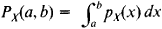# Random Variable

(redirected from Statistical variable)
Also found in: Dictionary, Thesaurus, Medical, Financial.

## random variable

[′ran·dəm ′ver·ē·ə·bəl]
(mathematics)
A measurable function on a probability space; usually real valued, but possibly with values in a general measurable space. Also known as chance variable; stochastic variable; variate.

## Random Variable

in probability theory, a quantity whose assumption of particular values is controlled by chance. Each of the values the random variable can assume has a certain probability. Thus, the number of spots on the top face of a die is a random variable that assumes the values 1, 2, 3,4, 5 and 6; the probability of each value is 1/6.

If a random variable X has a finite or infinite sequence of distinct values, the probability distribution function (distribution law) of X can be specified by indicating these values

x1, x2, …, xn, …

and the probabilities associated with these values

p1,, p2, …, pn, …

This type of random variable is said to be discrete. In other cases, the probability distribution function can be specified by indicating for each closed interval Δ = [a, b] the probability Px(a, b) of the inequality ax < b. Random variables are encountered particularly often for which there exists a function Px(x), called the probability density function, such thatThis type of random variable is said to be continuous.

A number of general properties of the probability distribution function of a random variable can be described sufficiently fully by a small set of numerical characteristics. The most frequently used characteristics are the mathematical expectation EX of the random variable X and the variable’s variance DX. Such characteristics as the median, mode, and quantile are less often used. (See alsoPROBABILITY THEORY.)

### REFERENCES

Gnedenko, B. V. Kurs teorii veroiatnostei, 5th ed. Moscow, 1969.
Cramer, H. Sluchainye velichiny i raspredeleniia veroiatnostei. Moscow, 1947. (Translated from English.)
Site: Follow: Share:
Open / Close